Középiskolai Matematikai és Fizikai Lapok
Informatika rovattal
 Már regisztráltál? Új vendég vagy?

# Exercises and problems in Physics October 1997

## New experimental problem:

m. 191. Measure the specific heat of the material of some coin.

## New exercises:

FGy. 3089. Why is less force required to slice a salami if the knife is moved forward and backward while cutting than if it is only pressed downward?

FGy. 3090. The battleship called VASA sunk near Stockholm was lifted out by means of steel tanks. The volume of each tank was 1500 m3, its mass was 300 tons. What lifting force could such a tank exert?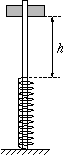FGy. 3091. A perforated body can move without friction on the vertical rod shown in the figure. When the body is carefully let onto a sufficiently long spring, the spring is compressed by 1 cm. From what height h should the body be dropped so that the largest compression of the spring is 8 cm?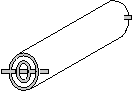FGy. 3092. A thin tube is pushed into a thicker one and fixed to it at both ends. The tubes are loosely in contact, equally long, but their materials are different. What is the longitudinal linear thermal expansion coefficient of this double tube? The cross-sections of the tubes are A1 and A2, the linear thermal expansion coefficients and Young's moduli are respectively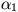and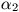, and E1 and E2.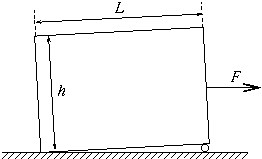FGy. 3093. A uniformly filled wooden box of mass M, width L and height h is pulled horizontally by means of a rope fastened to the centre of its side (see the figure). A cylindrical roller of small diameter is placed under this side of the box. The coefficient of friction between the box and the ground is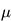, rolling friction is negligible. What force F is required to set the box into motion?

## New problems:

FF. 3094. Coulomb's law prescribes an infinitely large force to make two point-like charges of identical sign touch. What is the situation if the charges are uniformly distributed on two circular plates? What is the force required to make two circular plates of charge Q and radius r touch?

FF. 3095. 80 liters of gaseous oxygen at a pressure of 5.105 Pa and a temperature of 32 oC are compressed adiabatically, which raises the temperature to 78 oC. What amount of work is done?

FF. 3096. Estimate the maximum change in the volume of a football with a mass of 0.4 kg and an excess pressure of 5.104 Pa,volleyed at an angle of 30o and landing at a distance of 50 m.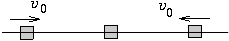FF. 3097. Three small bodies of identical masses can move along a straight line. The central body is initially at rest, then the two other bodies hit it nearly at the same moment and at the same speed. The collision of the body on the left is perfectly elastic, while that of the body on the right is perfectly inelastic. How do the bodies move after the collisions?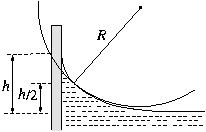FN. 3098. How high does water crawl up a smooth vertical wall if it adheres to the wall? What is the force exerted by the wall onto the water? What is the radius of curvature of the surface of the water at half of the maximum height (in the plane perpendicular to the wall)?